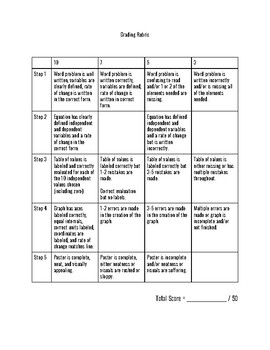# Writing & Graphing Linear EquationsSubject
Resource Type
File Type

PDF

(175 KB|3 pages)
Standards
• Product Description
• StandardsNEW

This project is designed to teach middle school students the basics of direct variation, constants of proportionality, writing linear equations, creating tables, and transposing their information onto a coordinate plane.

This also encourages group work, creativity, and ingenuity as students will create their own, personal word problems, and then translate their verbal phrases into equations, tables, and graphs.

The third page of this PDF is also the grading rubric!

Use variables to represent two quantities in a real-world problem that change in relationship to one another; write an equation to express one quantity, thought of as the dependent variable, in terms of the other quantity, thought of as the independent variable. Analyze the relationship between the dependent and independent variables using graphs and tables, and relate these to the equation. For example, in a problem involving motion at constant speed, list and graph ordered pairs of distances and times, and write the equation 𝘥 = 65𝘵 to represent the relationship between distance and time.
Solve real-world and mathematical problems by writing and solving equations of the form 𝘹 + 𝘱 = 𝘲 and 𝘱𝘹 = 𝘲 for cases in which 𝘱, 𝘲 and 𝘹 are all nonnegative rational numbers.
Use variables to represent numbers and write expressions when solving a real-world or mathematical problem; understand that a variable can represent an unknown number, or, depending on the purpose at hand, any number in a specified set.
Understand solving an equation or inequality as a process of answering a question: which values from a specified set, if any, make the equation or inequality true? Use substitution to determine whether a given number in a specified set makes an equation or inequality true.
Total Pages
3 pages
Rubric only
Teaching Duration
2 days
Report this Resource to TpT
Reported resources will be reviewed by our team. Report this resource to let us know if this resource violates TpT’s content guidelines.